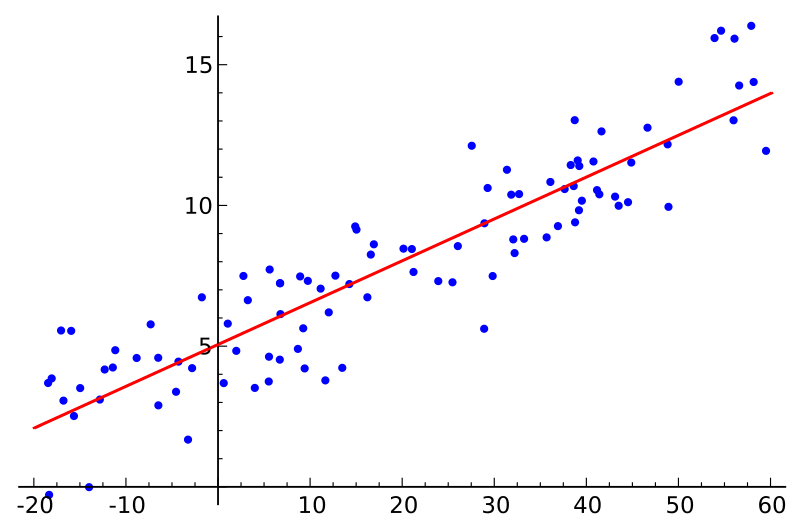## Simple linear regression in DAXDAX, originating in Power Pivot, shares many functions with Excel. As of 2017, some of the functions, such as SLOPE and INTERCEPT, exist in the latter but not in the former. The two functions can be used for a simple linear regression analysis, and in this article I am sharing patterns to easily replicate them in DAX. But first, why would you want to do such analysis? Continue reading “Simple linear regression in DAX”